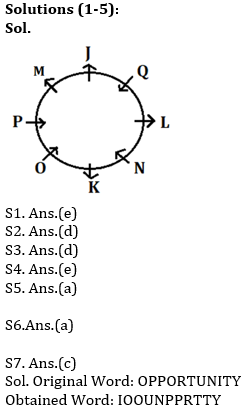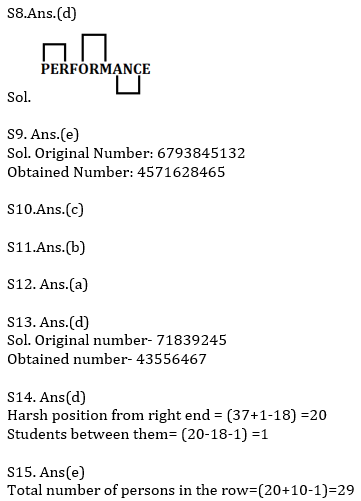Latest Banking jobs   »   Reasoning Ability Quiz For IBPS Clerk/NIACL...

# Reasoning Ability Quiz For IBPS Clerk/NIACL AO Prelims 2021- 29th September

Directions (1-5): Study the information and answer the given questions:
Eight persons- J, K, L, M, N, O, P and Q are sitting around a circular table. Some of them are facing inside the center while some are facing outside the center. L sits third to the right of O who is facing inside the center. K sits 2nd to the right of L. K sits third to the left of Q. J sits second to the left of L. Both P and M sits immediate left to each other. Only two persons sit between J and N. K faces same direction as M but opposite to Q. P sits second to the left of J. P and N facing same direction but opposite to L.

Q1. Who among the following sit opposite to the one who sits 3nd left of M?
(a) L
(b) Q
(c) M
(d) K
(e) None of these

Q2. Who among the following faces O?
(a) J
(b) K
(c) M
(d) Q
(e) P

Q3. How many persons sits between L and O when counted in clockwise direction with respect to O?
(a) One
(b) Three
(c) Two
(d) Four
(e) No one

Q4. If Q is related to N and L is related to J, then M is related to whom among the following?
(a) N
(b) K
(c) P
(d) J
(e) Q

Q5. Who among the following sits immediate right of N?
(a) L
(b) M
(c) Q
(d) K
(e) O

Q6. Which of the following elements should come in a place ‘?’ ?
GH1 IK2 LO3 PT4 ?
(a) UZ5
(b) VZ5
(c) UX5
(d) VX5
(e)None of these

Q7. If all the letters in the word OPPORTUNITY are arranged in alphabetical order from left to right in such a way that vowels are arranged first followed by consonants, then how many letters are there in between I and R after the arrangement?
(a) None
(b) Two
(c) More than Five
(d) Five
(e) Four

Q8.How many pairs of letters are there in the word ‘PERFORMANCE’, each of which have as many letters between then in the word as they have between then in the English alphabet?
(a) More than Three
(b) Four
(c) One
(d) Three
(e) None

Q9. If in the number 6793845132, 2 is subtract to first six digits of the number and 3 is added in rest of digits in the number, then how many digits are repeating in the number thus formed?
(a) Two
(b) None
(c) One
(d) Four
(e) Three

Q10. Which alphabet is 3rd from the left in the meaningful four-letter word formed from the first, third, seventh and Eighth letter of the word Population? If more than one word is formed, then mark answer as X and no meaningful word is formed then mark answer as Z.
(a) T
(b) X
(c) Z
(d) P
(e) I

Q11.Pointing to a Lady, A Man said, “She is the maternal aunt of my father’s only daughter” How is the daughter of Man’s father is related to Man?
(a) Herself
(b) Sister
(c) Daughter
(d) Either (a) or (b)
(e) Father

Q12. Himani says pointing towards a Girl, “She is the daughter of the wife of my brother”. In this relation, Himani is a female then how Himani is related to the girl?
(a) Aunt
(b) Father
(c) Uncle
(d) Grandfather
(e) None of these

Q13. If in the number 71839245, 3 is subtracted to each of the digit which is greater than 6 and 2 is added from each of the digit which is less than 6, then which of the following digit is second to the right of the digit which is third from the left end?
(a) 3
(b) 7
(c) 4
(d) 6
(e) 5

Q14. Harsh is 18th from the left end of a row of 37 students and Reena is 18th from the right end in the same row. How many students are there between them in the row?
(a) 2
(b) 3
(c) 5
(d) 1
(e) 4

Q15. Sameer is 20th from the left end of a row and Arun is 12th from the right end of row. If they interchanged their positions, then arun ranks become 10 from right end. Find total number of persons in the row?
(a) 30
(b) 39
(c) 28
(d) 31
(e) None of these

SolutionsClick Here to Register for Bank Exams 2021 Preparation Material#### Congratulations!Download Hindu Review of October 2021: Free PDF